# 4D Visualization

## Rotations (1)

We continue our exploration of the 4D world by taking a look at rotations.

### Rotation being a Planar Phenomenon

One peculiarity of the 3D space that we inhabit is that rotation has a unique axis around which every point revolves. The points that lie on this axis are stationary under the rotation; so we may call the axis the stationary line of the rotation.

Since our experience is limited to 3D space, we may be tempted to think that rotational axes are an inherent property of rotation. However, this is actually not true of other dimensions in general. Consider the following square rotating in the 2D plane: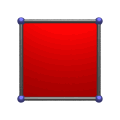Where is the axis for this rotation? From our 3D viewpoint, it lies perpendicular to the 2D plane, protruding outward from the screen. However, if we were to look from the 2D perspective, there is no such axis; there is only a single stationary point at the center of the rotation. All other points on the supposed axis lie outside the 2D universe!

As we shall see, rotations in 4D do not have unique axes of rotation. Instead, they have stationary planes which remain stationary under the rotation. From the 4D perspective, a rotation has not just an axis, but a plane around which everything else rotates. As 3D beings, we may find it difficult to understand just how something could rotate “around” a plane.

Instead of thinking about stationary points, axes, or planes, it is much better to understand rotations directly, as a planar phenomenon. Consider 2D and 3D rotations, which we are acuainted with. There is one common characteristic among them: every point of the rotating object traces out circles that lie on parallel planes. In 2D, there is only one such plane, the 2D universe itself, whereas in 3D, there may be many parallel planes. Since they must always be parallel (otherwise it is not a rotation, but a deformation of the object), we can pick one such representative plane and call it the plane of rotation. Thus, we may think of rotation as happening in a plane rather than around an axis.

This understanding of rotation is easier to generalize to higher dimensions. It also helps us understand why 2D rotations only have stationary points, whereas 3D rotations have axes: being a planar phenomenon, a rotation “needs” two dimensions to move in. In 2D, these are the only two dimensions available; hence what is left is only a 0D stationary point. In 3D, there are 3 available dimensions, so there is still 1 dimension left when the other two are employed for the rotation. This extra dimension corresponds with a single line, the rotational axis. In 4D, there are 2 dimensions left over, and so we have stationary planes.

### Number of Principal Rotations

Those of us who are geometrically-inclined would no doubt have learned that there are three principal rotations in 3D: those in the XY, YZ, and XZ planes. Every rotation in 3D can be reduced to a combination of these principal rotations. The fact that there are precisely 3 principal rotations in 3D, however, is a coincidence: the number of principal rotations is, in general, not equal to the number of dimensions. For example, in 2D, there is only one plane of rotation, the 2D plane itself. As we shall see, the number of principal rotations in 4D is not four, but six.

This is a simple matter of combinatorics: we have already seen that rotations are a planar phenomenon, and therefore “use up” two dimensions. The number of different principal rotations, therefore, is equal to the number of distinct pairs of dimensions. For example, rotation is not possible in 1D, because there is only one axis, the X-axis, and no pairs are possible. In 2D, there are two axes: X and Y, and these are the only possible pair of dimensions; therefore, there is precisely 1 plane of rotation. In 3D, there are three axes: X, Y, and Z; this gives us 3 possible pairs: XY, XZ, and YZ. Hence, there are 3 principal rotations in 3D. In 4D, we have four axes, X, Y, Z, and W; so there are six possible pairs: XY, XZ, XW, YZ, YW, and ZW. (It is left as an exercise to the reader to check that these are the only possibilities.)

The reader may feel a little overwhelmed at this point: there are six principal rotations in 4D to be learned, twice the number in 3D! However, this is actually not as hard as it may seem at first. The key here is to visualize 4D rotations using projections.

### The Appearance of Projected Rotations

Consider how one might teach 3D rotations to a 2D creature. One useful way would be to use a projection along the Z axis, so that the X and Y axes of 3D space map to the X and Y axes of 2D, and the Z axis is collapsed into a point. Here is what a square rotating in the XZ plane might look like under this projection: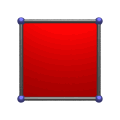For us 3D beings, it is easy to immediately interpret this in the 3D sense; but think for a moment from a purely 2D perspective. Remember that the 2D being can only see a 1D projection of this image. From its point of view, instead of a square rotating in the plane (which is the only type of rotation it would be acquainted with), it sees a strange morphing object turning itself inside-out in an impossible contortion. It would be difficult to believe that such a strange object is a square. Of course, we know from our 3D perspective that the square isn't really morphing; the rotation just appears that way because it is seen from the side.

What about the appearance of a square rotating in the YZ plane?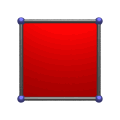Again, we see the “turning inside-out” effect. This rotation appears to be identical to the first one, except that the “inside-out” effect takes place along the Y-axis rather than the X-axis. Finally, let's look at the last principal 3D rotation, the one in the XY plane:Notice how this rotation looks exactly like a 2D rotation. This should not be surprising, since rotation in the XY plane happens to be the same as the only principal rotation in 2D.

So here we see that projections of 3D rotations into 2D can appear in two possible ways: as a perfectly normal 2D rotation, or as an apparent “turning inside-out”. The normal-looking rotation is the one in the XY plane, which happens to be the same as the principal 2D rotation. The “inside-out” rotations are the ones that happen in planes involving the Z-axis, the axis that extends outside the 2D plane. Furthermore, these “inside-out” rotations are identical in appearance to each other except for orientation.

### Visualizing 4D Rotations

The same thing happens with 4D rotations: 3 of the 6 possible principal rotations appear exactly like the 3 principal 3D rotations that we are already familiar with. They are the rotations in the XY, XZ, and YZ planes, which happen to be the same as the 3 principal rotations in 3D. The remaining rotations are the ones involving the W-axis: the rotations in the XW, YW, and ZW planes. They have an appearance of “turning inside-out”. The following animations show a cube undergoing each respective rotation: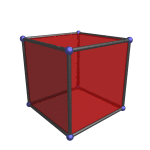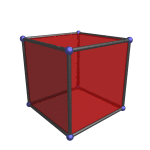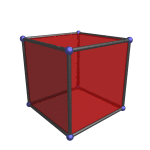Notice that all three rotations appear the same, except that the “turning inside-out” happens along the X-, Y-, and Z-axes respectively. Hence, we really only need to learn one new type of rotation, and we will have fully grasped all 6 principal rotations in 4D.

It is important to keep in mind that in all of these animations, the cube is not actually distorting and turning inside-out. This is merely an artifact of projecting from 4D to 3D. The cube retains its shape unchanged throughout all of the rotations.

### Clifford Rotations

We said earlier that rotation requires 2 dimensions, and mentioned that the reason 4D rotations have stationary planes is because there are 2 dimensions left over. The perceptive reader may have wondered if it was possible to have a second, distinct rotation in this stationary plane, since there are 2 leftover dimensions for another rotation to happen in. That is to say, is it possible for an object to rotate simultaneously around the XY plane and the ZW plane, possibly with two different rates of rotation, since the rotational plane of each lies precisely in the stationary plane of the other?

The answer is, in fact, yes. It is quite possible for an object in 4D to rotate simultaneously in the XY and ZW planes—or, for that matter, the XZ and YW planes, or the YZ and XW planes. These complex gyrations are known mathematically as Clifford rotations (or double rotations). To distinguish these from the rotations we considered earlier, we shall refer to the former as plane rotations. Clifford rotations can be decomposed into two independent, simultaneous plane rotations (hence the name double rotation), and therefore have two independent rates of rotation.

Mathematicians have known of Clifford rotations for a long time, although not many can easily visualize them, since they are not possible in dimensions below 4. With what we have learned so far, however, we are ready to actually see a 4D Clifford rotation in action. It is simply a rotation where, in projection, a 3D-like rotation happens at the same time as an “inside-out” rotation. For example, here is a cube rotating simultaneously in the XY and ZW planes: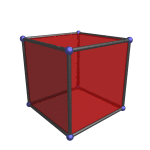The “inside-out” rotation is happening along the vertical Z-axis, which corresponds with the ZW plane, and the 3D-like rotation is happening in the XY plane. Notice how the ZW plane projects onto the Z-axis, which is the axis of the XY rotation in 3D.

A 4D Clifford rotation does not have a stationary plane, since all 4 dimensions are being used for the rotation. Instead, it has a stationary point, which is the intersection of the stationary planes of its two constituent plane rotations. (In 4D, two planes will generally intersect only at a point.) In this particular case, the stationary point of the cube lies at the center of the cube.

Last updated 02 Feb 2023.